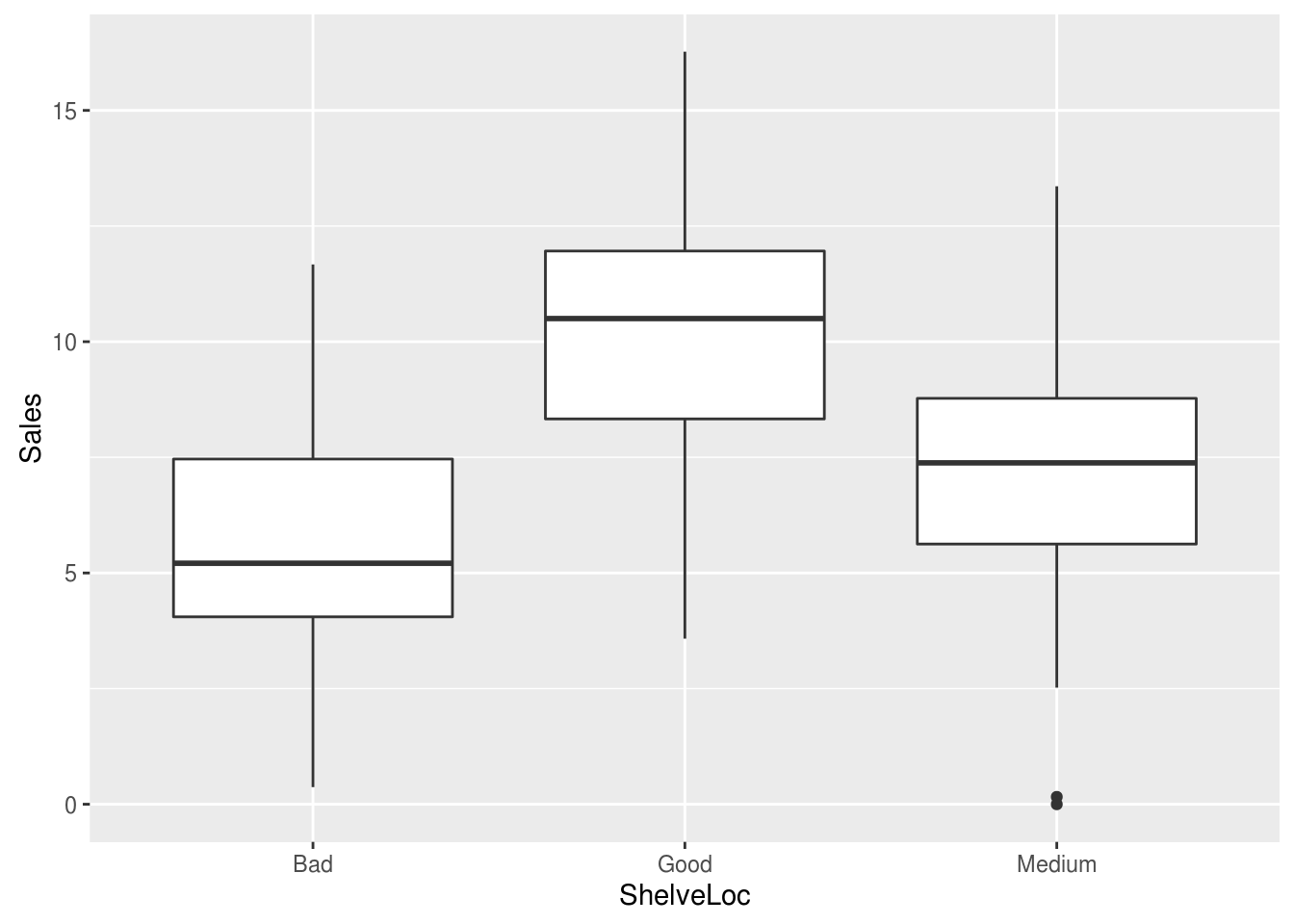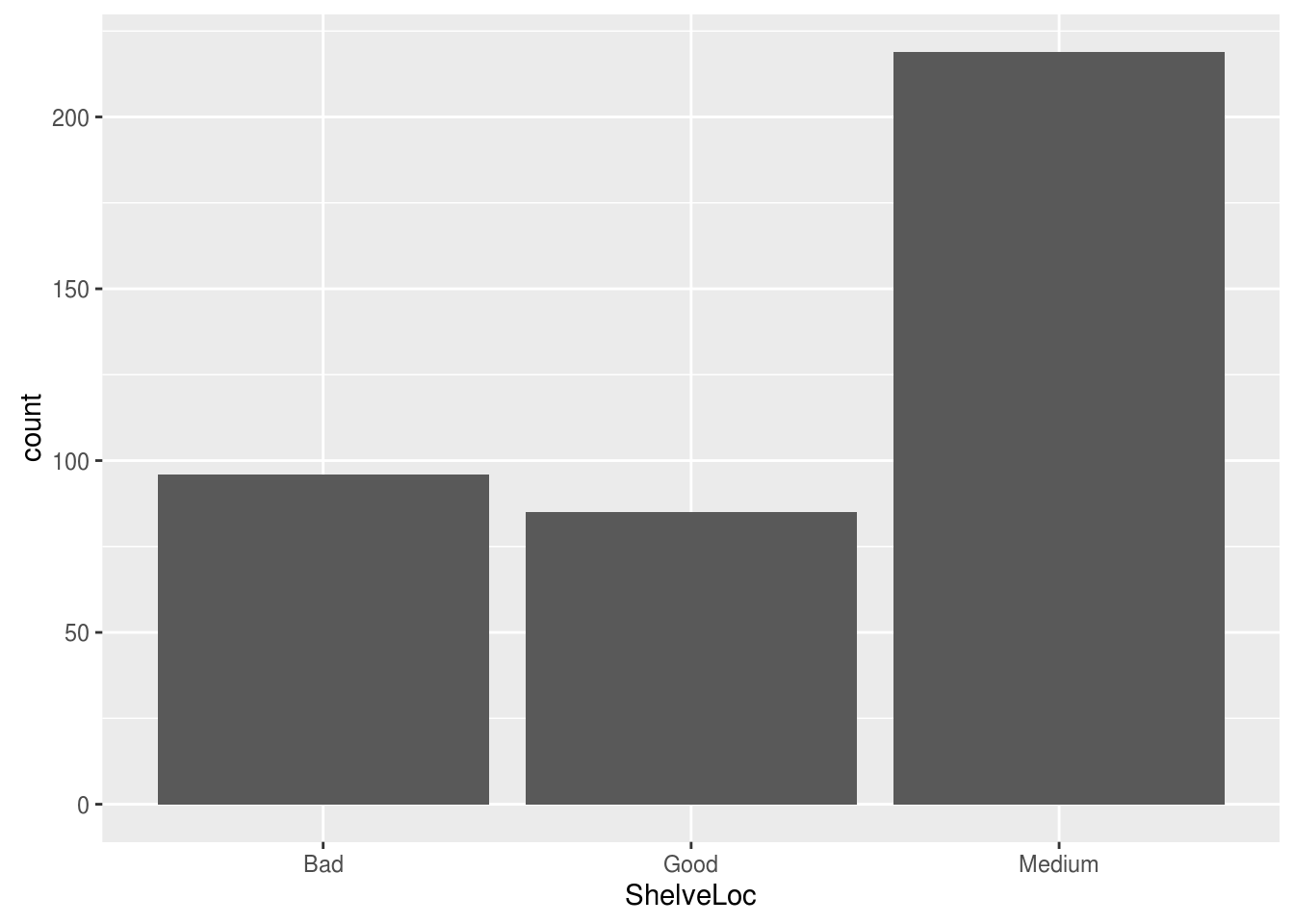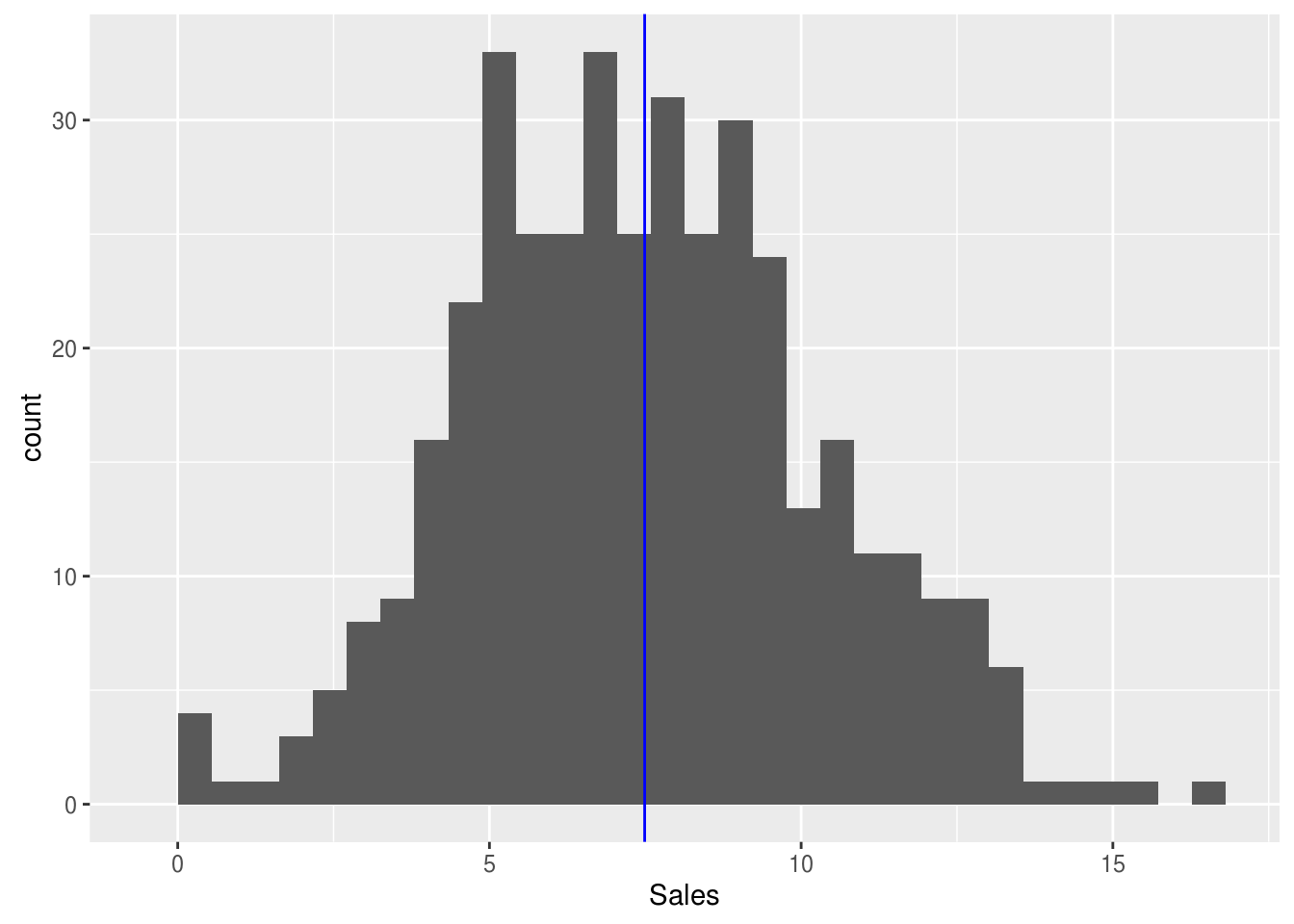# Using Qplots for Graphs in R

In this post, we will explore the use of the “qplot” function from the “ggplot2” package. One of the major advantages of “ggplot” when compared to the basegraphics package in R is that the “ggplot2” plots are much more visually appealing. This will make more sense when we explore the grammar of graphics. for now, we will just make plots to get used to using the “qplot” function.

We are going to use the “Carseats” dataset from the “ISLR” package in the examples. This dataset has data about the purchase of carseats for babies. Below is the initial code you will need to make the various plots.

``````library(ggplot2);library(ISLR)
data("Carseats")``````

In the first scatterplot, we are going to compare the price of a carseat with the volume of sales. Below is the code

``qplot(Price, Sales,data=Carseats)``Most of this coding format you are familiar. “Price” is the x variable. “Sales” is the y variable and the data used is “Carseats. From the plot, we can see that as the price of the carseat increases there is normally a decline in the number of sales.

For our next plot, we will compare sales based on shelf location. This requires the use of a boxplot. Below is the code

``qplot(ShelveLoc, Sales, data=Carseats, geom="boxplot")``The new argument in the code is the “geom” argument. This argument indicates what type of plot is drawn.

The boxplot appears to indicate that a “good” shelf location has the best sales. However, this would need to be confirmed with a statistical test.

Perhaps you are wondering how many of the Carseats were in the bad, medium, and good shelf locations. To find out, we will make a barplot as shown in the code below

``qplot(ShelveLoc, data=Carseats, geom="bar")``The most common location was medium with bad and good be almost equal.

Lastly, we will now create a histogram using the “qplot” function. We want to see the distribution of “Sales”. Below is the code

``qplot(Sales, data=Carseats, geom="histogram")``
``## `stat_bin()` using `bins = 30`. Pick better value with `binwidth`.``The distribution appears to be normal but again to know for certain requires a statistical test. For one last, trick we will add the median to the plot by using the following code

``qplot(Sales, data=Carseats, geom="histogram") + geom_vline(xintercept = median(Carseats\$Sales), colour="blue")``
``## `stat_bin()` using `bins = 30`. Pick better value with `binwidth`.``To add the median all we needed to do was add an additional argument called “geom_vline” which adds a line to a plot. Inside this argument, we had to indicate what to add by indicating the median of “Sales” from the “Carseats” package.

Conclusion

This post provided an introduction to the use of the “qplot” function in the “ggplot2” package. Understanding the basics of “qplor” is beneficial in providing visually appealing graphics